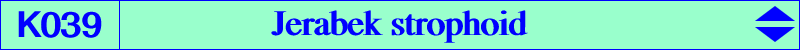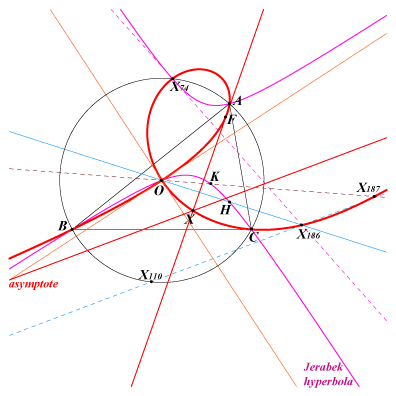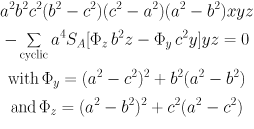X(3), X(74), X(186), X(187), X(1157), X(3455), X(5172), X(5866), X(5961), X(6091), X(11589), X(12011), X(13754), X(15469), X(15478), X(18876), X(34418), X(34442), X(39986), X(40047), X(40076), X(40077), X(40078), X(40079), X(40080), X(40081), X(40082), X(40083), X(40084) other points belowThe inversive image (in the circumcircle) of the Jerabek hyperbola is the Jerabek strophoid. It is also the isogonal transform of the Ehrmann strophoid K025. K025 and K039 are members of the class CL038 of non-isogonal circum-strophoids. See also Q038. The node of K039 is O = X(3) with two tangents parallel to the asymptotes of the Jerabek hyperbola. The singular focus F is X(5961), the inverse of X(265) in the circumcircle. Recall that X(265) is the antipode of X(3) on the Jerabek hyperbola. This strophoid is, with the line at infinity, the locus of P such that the reflections of AP, BP and CP through AO, BO and CO are concurrent in a point P* i.e. P and P* can be reflected via A, B, C in the circumcircle as circular mirror. Notice that P and P* can also be reflected via X(74) in the circular mirror (Floor van Lamoen).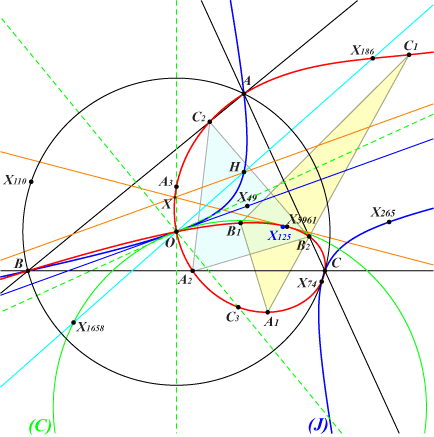P and P* are conjugate points on K039 and their inverses in (O) are two antipodes on the Jerabek hyperbola (J). In other words, if iM is the inverse of M in (O) and rM is the reflection of M in the center X(125) of (J) then P* = iriP = f(P). According to known properties of focal cubics, the midpoint of PP* lies on the orthic line of the cubic which is the tangent at O to (J). This line passes through X(49). The real asymptote of K039 is the homothetic of this latter line under h(X5961, 2). Its infinite point is X(13754) = gX(1300) and its intersection X with the cubic is X(40047). Hence, K039 contains the following points : • A1 = f(A), B1 and C1 likewise, • A2 = BC /\ B1C1, B2 and C2 likewise, • A3 = f(A2), B3 and C3 likewise.Remarks : • A3 lies on the perpendicular bisector of BC, • the triangles A1B1C1 and A2B2C2 are perspective at X(110), • the triangles ABC and A3B3C3 are orthologic at X(3) and X(24).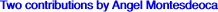In the reference triangle ABC, let P be a point and DEF the circumcevian triangle of P. Ab is the center of the circle passing through D and tangent to AB at B. Ac is the center of the circle passing through D and tangent to AC at C. Similarly define Bc, Ba and Ca, Cb. The triangle A'B'C' bounded by the  lines AbAc, BcBa, CaCb is not degenerate and perspective to ABC if and only if P lies on the Jerabek strophoid (Angel Montesdeoca, private message 2014-05-08). Note that ABC and A'B'C' are also orthologic. These triangles are parallelogic if and only if P lies on the focal cubic nK(X571, X1994, X110) whose singular focus is the same as that of K039 i.e. F as above.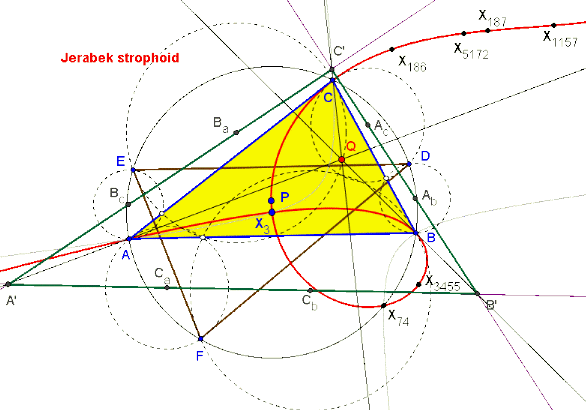Given a triangle ABC and a point P, the parabola with focus P and directrix BC intersects the A-altitude again at Pa. The points Pb and Pc are defined cyclically. When P traverses the nine-point circle, ABC and PaPbPc are orthologic at H and another point P' that lies on K039 (Angel Montesdeoca, private message 2023-01-16).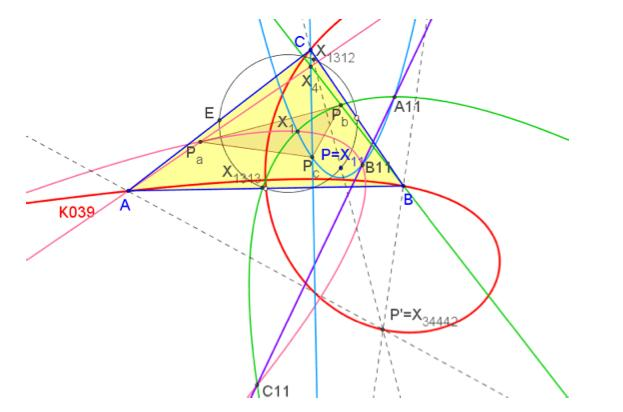If P(u:v:w) then P' =(a^2 u)/((b^2 - c^2)^2 (u + v + w)^2 + a^4 (-3 u^2 + (v - w)^2 - 2 u (v + w)) + 2 a^2 (c^2 (u^2 + v^2 - w^2) + b^2 (u^2 - v^2 + w^2))) : : . Pairs {P,P'}, with P on the nine-point circle and P' on K039 : {11,34442}, {113,15469}, {114,40083}, {115,3455}, {116,40076}, {120,40084}, {122,40082}, {124,40081}, {125,74}, {126,40078}, {127,18876}, {131,40047},{132,40080}, {135,15478}, {136,5961}, {137,34418}, {1312,3}, {1313,3}, {2679,40077},{3258,186}, {5099,187}, {5139,6091}, {5520,5172}, {16177,11589}, {16221,13754},{25641,39986}, {42426,40079}, {46439,1157}, {48317,5866}. P' is the intersection of the parallel at O to the line P, X(113) and the line passing through X(186) and the reflection of H in P. Other pairs {P,P'} : {4, 3}, {5095, 895}, {20411, 51243}, {20412, 51242}.